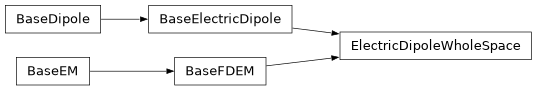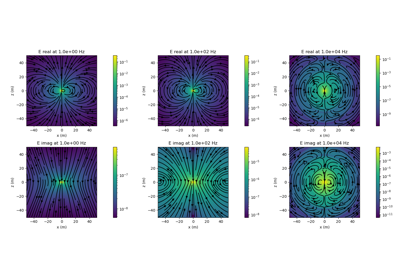# geoana.em.fdem.ElectricDipoleWholeSpace#class geoana.em.fdem.ElectricDipoleWholeSpace(frequency, quasistatic=False, **kwargs)#

Class for simulating the fields and fluxes for a harmonic electric dipole in a wholespace.

Harmonic electric dipole in a whole space. The source is (c.f. Ward and Hohmann, 1988 page 173). The source current density for a dipole located at $$\mathbf{r}_s$$ with orientation $$\mathbf{\hat{u}}$$

$\mathbf{J}(\mathbf{r}) = I ds \delta(\mathbf{r} - \mathbf{r}_s)\mathbf{\hat{u}}$

Attributes

 current Current in the electric current dipole (A) epsilon Dielectric permittivity in F/m frequency Frequency (Hz) used for all computations length Length of the electric current dipole (m) location Location of the dipole mu Magnetic permeability in H/m omega Angular frequency orientation Orientation of the dipole as a normalized vector sigma Electrical conductivity in S/m sigma_hat Conductivity including electric displacement term. skin_depth Returns the skin depth for an electromagnetic wave in a homogeneous isotropic medium. wavenumber Wavenumber for an electromagnetic planewave in a homogenous isotropic medium.

Methods

 Cross products between a gridded set of vectors and the orientation of the source. Current density for the harmonic current dipole at a set of gridded locations. distance(xyz) Scalar distance from dipole to a set of gridded xyz locations Dot product between the orientation of the source and a gridded set of vectors. Electric field for the harmonic current dipole at a set of gridded locations. Magnetic field produced by the harmonic current dipole at a set of gridded locations. Magnetic flux density produced by the harmonic electric current dipole at a set of gridded locations. Vector distance to a set of gridded xyz locations. Vector potential for the harmonic current dipole at a set of gridded locations.

## Galleries and Tutorials using geoana.em.fdem.ElectricDipoleWholeSpace#Electric Dipole in a Whole Space: Frequency Domain

Electric Dipole in a Whole Space: Frequency Domain